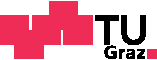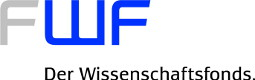## Integer-valued Polynomials (FWF-grant P23245-N18)### Project Summary

In the classical sense, integer-valued polynomials are polynomials with rational coefficients that map all integers to integers. Already in the 17th century, Newton used this kind of polynomials to interpolate functions. At the beginning of the 20th century, Pólya, Ostrowski and Skolem discovered their role in number theory.
In addition to their usefulness for interpolation, integer-valued polynomials have other interesting algebraic and number theoretic properties. For example, they satisfy a $p$-adic version of the Stone-Weierstrass property which make them suitable for approximation purposes, and analogues of Hilbert's Nullstellensatz, the so-called Skolem properties.
We researchers of this project, Sophie Frisch, Giulio Peruginelli and Roswitha Rissner, worked in the more general context of integer-valued polynomials on arbitrary domains. We narrowed down the gap between sufficient and necessary conditions for Skolem properties to almost nothing, the first significant advance on this question in decades.
Also, we were among the first to investigate polynomials which are integer-valued on matrix rings or more general algebras. We showed that integer-valued polynomials with coefficients in a non-commutative matrix algebra can be expressed as matrices whose entries are polynomials with coefficients in a commutative ring. In the special case of upper triangular matrix algebras, integer-valued polynomials coincide with polynomials whose divided differences up to order $n$ are integer-valued. These polynomials have applications, discovered by Bhargava, to Banach spaces of $n$-times continuously differentiable functions on a compact subset of a local field.
We also showed various other number theoretic and ring theoretic results, well-received by the international scientific community, concerning integer-valued polynomials, their factorization, their overrings and the Prüfer property.

### Researchers

The mathematicians supported by the FWF grant P2345-N18 are:

### Events

We organized the Workshop and Conference on

Commutative Ring Theory, Integer-valued Polynomials and Polynomial Functions

in Graz, Austria in December 2012 at Graz University of Technology.

### Proceedings

On the occasion of the conference in December 2012, we published proceedings with Springer:

Commutative Algebra, Recent Advances in Commutative Rings, Integer-Valued Polynomials, and Polynomial Functions, Springer New York, 2014.

### Contact

Sophie Frisch
Graz University of Technology
Department of Analysis und Number Theory (Math A)
Kopernikusgasse 24, NT02022
8010 Graz, Austria
frisch (at) blah.math.tugraz.at

Last edited: May 2016 by Roswitha Rissner